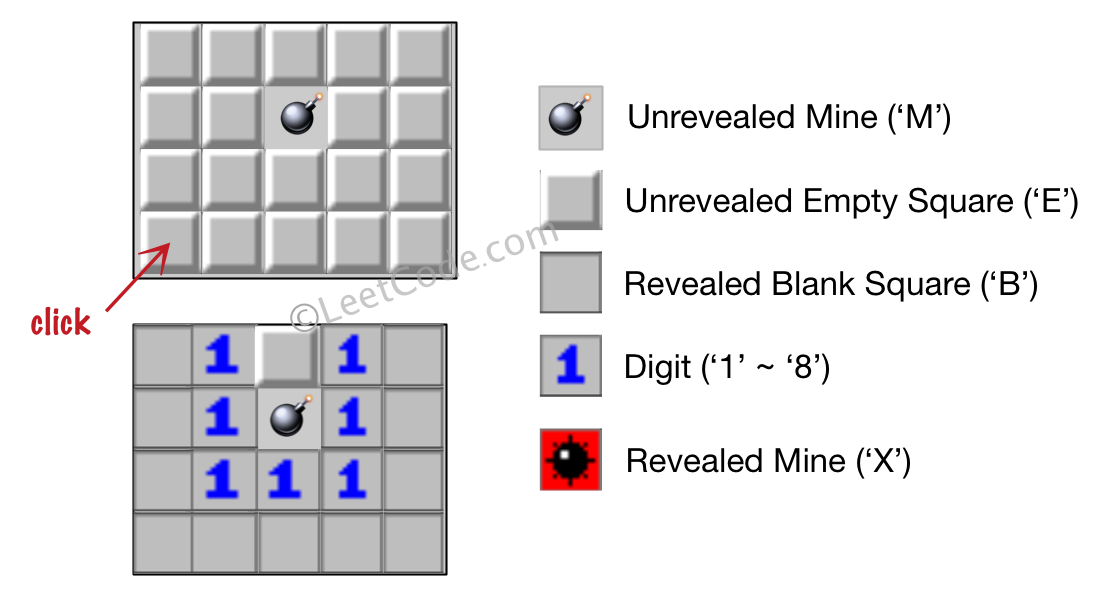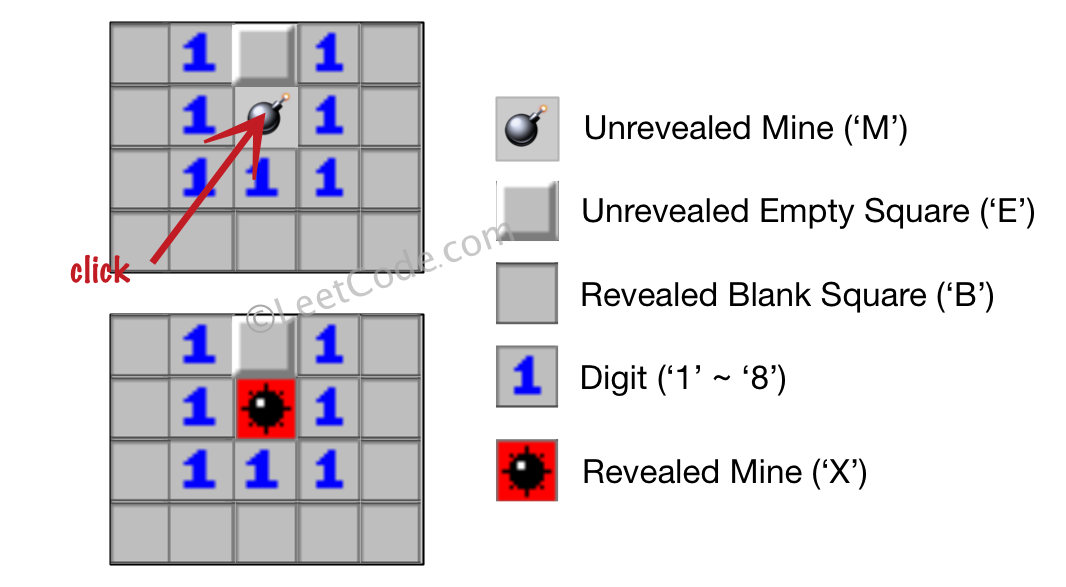# LeetCode Minesweeper Solution

Let's play the minesweeper game (Wikipedia, online game)!

You are given an `m x n` char matrix `board` representing the game board where:

• `'M'` represents an unrevealed mine,
• `'E'` represents an unrevealed empty square,
• `'B'` represents a revealed blank square that has no adjacent mines (i.e., above, below, left, right, and all 4 diagonals),
• digit (`'1'` to `'8'`) represents how many mines are adjacent to this revealed square, and
• `'X'` represents a revealed mine.

You are also given an integer array `click` where `click = [clickr, clickc]` represents the next click position among all the unrevealed squares (`'M'` or `'E'`).

Return the board after revealing this position according to the following rules:

1. If a mine `'M'` is revealed, then the game is over. You should change it to `'X'`.
2. If an empty square `'E'` with no adjacent mines is revealed, then change it to a revealed blank `'B'` and all of its adjacent unrevealed squares should be revealed recursively.
3. If an empty square `'E'` with at least one adjacent mine is revealed, then change it to a digit (`'1'` to `'8'`) representing the number of adjacent mines.
4. Return the board when no more squares will be revealed.

Example 1:Input: `board = [["E","E","E","E","E"],["E","E","M","E","E"],["E","E","E","E","E"],["E","E","E","E","E"]], click = [3,0]`
Output: `[["B","1","E","1","B"],["B","1","M","1","B"],["B","1","1","1","B"],["B","B","B","B","B"]]`

Example 2:Input: `board = [["B","1","E","1","B"],["B","1","M","1","B"],["B","1","1","1","B"],["B","B","B","B","B"]], click = [1,2]`
Output: `[["B","1","E","1","B"],["B","1","X","1","B"],["B","1","1","1","B"],["B","B","B","B","B"]]`

Constraints:

• `m == board.length`
• `n == board[i].length`
• `1 <= m, n <= 50`
• `board[i][j]` is either `'M'`, `'E'`, `'B'`, or a digit from `'1'` to `'8'`.
• `click.length == 2`
• `0 <= clickr < m`
• `0 <= clickc < n`
• `board[clickr][clickc]` is either `'M'` or `'E'`.

## Solution

There are a few things we need to keep in mind when implementing the problem:

• blank squares (`'B'`) and digit squares (`'1'`-`'8'`) should be treated differently
• a blank square's neighbours need to be revealed recursively
• only recursively reveal empty squares ('E')

We are given the `click` coordinate to update the board. If `click` is a mine (`'M'`), then we change it to `'X'`. If it's an empty square, then the `update` function updates the empty square recursively.

#### Implementation

``````1def updateBoard(board: List[List[str]], click: List[int]) -> List[List[str]]:
2    if board == []: return []
3    row = len(board)
4    col = len(board)
5
6    def isbomb(i, j):
7        if i < 0 or j < 0 or i >= row or j >= col:
8            return False
9        elif board[i][j] == 'M' or board[i][j] == 'X':
10            return True
11        else: return False
12
13    def update(r, c):
14        if r < 0 or c < 0 or r >= row or c >= col: return
15        if board[r][c] == 'E':    # empty square
16            bombs = 0             # number of neighbouring bombs
17            for i in range(r-1, r+2):
18                for j in range(c-1, c+2):
19                    if isbomb(i, j): bombs += 1
20            if bombs:             # update cell with number of bombs
21                board[r][c] = chr(48 + bombs)
22            else:                 # blank cell (0 bombs)
23                board[r][c] = 'B'
24                for i in range(r-1, r+2): # update neighbours
25                    for j in range(c-1, c+2):
26                        update(i, j)
27        # else already revealed, do nothing
28
29    r, c = click, click
30    if board[r][c] == 'M':  # bomb
31        board[r][c] = 'X'
32    else:                   # empty square, or revealed square
33        update(r, c)
34    return board``````